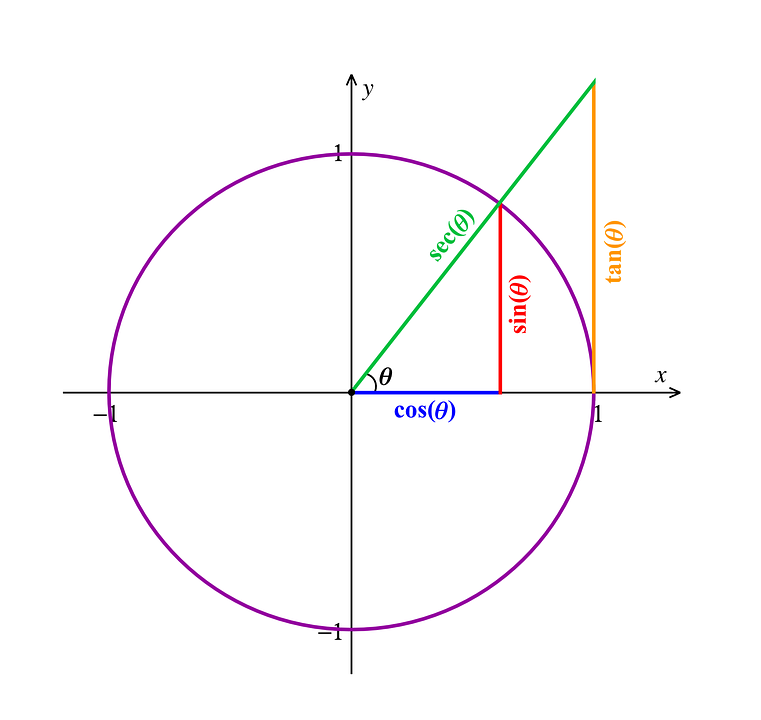# Cosine Formula

Did you know, that every straight-sided shape in geometry can be broken into triangles? This essentially is the basis of trigonometry. In this article, we will discuss the important cosine formula with proof and examples. Let us begin the topic!

Table of content

## Cosine Formula

Trigonometry is a very interesting branch of mathematics that studies relationships between the sides and angles of triangles. Trigonometry is found all throughout the geometry because every straight-sided shape may be broken into as a collection of triangles. Also, trigonometry has astoundingly intricate relationships with other branches of mathematics like in complex numbers, infinite series, logarithms, and calculus. The solution for an oblique triangle can be done with the important sine formula and cosine formula of the trigonometry.### What is the Cosine Formula?

Let us take an oblique triangle, i.e. a triangle with no right angle. Therefore, it is a triangle whose angles are all acute or a triangle with one obtuse angle. It is most useful for solving for missing information in a given triangle. For example, if all three sides of the triangle are known, then cosine formula will allow us to find any or all of its three angles. Similarly, if two sides and the angle between these two sides is known, then the cosine formula allows us to find the third side length.

The Cosine formula is used in the following cases:

1. If two sides and an included angle are given i.e. SAS
2. If three sides are given i.e. SSS

The Cosine formula, for a triangle with side lengths as a,b and c and angles $$\angle A, \angle B, \angle C$$ is given as :

(1) $$a^2= b^2+c^2-2bc\;cosA$$

(2) $$b^2= a^2+c^2-2ac\;cosB$$

(3) $$c^2= a^2+b^2-2ab\; cosC$$

Above formulas can also be written as:

(1) $$cosA = \frac{b^2+c^2-a^2}{2bc}$$

(2) $$cosB = \frac{a^2+c^2-b^2}{2ac}$$

(3) $$cosC = \frac{a^2+b^2-c^2}{2ab}$$

Obviously it can be seen as the general form of the Pythagorean theorem. Take any one side of the triangle, for instance, here ‘a’. If we have the $$\angle A as 90^{\circ}$$, then we would be left with the Pythagorean theorem. It is because

$$cos 90^{\circ} =0$$

Then we will have :

$$a^2 = b^2 + c^2$$

### Cosine Formula Derivation

We will prove the above formula for the side ‘a’. The other two equations can be easily proved in a similar way.

Proof: We will prove the above formula for the side ‘a’. Let’s denote its facing angle as $$\angle A$$. Let us draw a perpendicular on a base of length ‘b’, and its height length is’h’.

This perpendicular is dividing the base into two parts of length ‘r’ and ‘b-r’ respectively.

Thus,

$$sin A =\frac{h}{c}$$

i.e.$$\; h=c\;sinA$$

also$$\; cos \;A=\frac{r}{c}$$

i.e.$$\; r= c\; cos A$$

using Pythogorean theorem, in the triangle we have,

$$a^2=h^2+(b-r)^2$$

Putting the values of h and r in abobve equation we get,

$$a^2= (c\;sinA)^2+(b-c\;cosA)^2$$

i.e. $$\; c^2\;sin^2A+b^2-2bc\;cosA + c^2\;cos^2A$$

i.e. $$c^2(sin^2A +cos^2A)+b^2-2bc\;cos A$$

since $$\; sin^2A +cos^2A = 1$$

so, $$\; a^2= b^2+c^2-2bc\;cosA$$

Hence proved.

## Solved Example

Q: If in a triangle if lengths of two sides are 14cm and 10cm with $$44^{\circ}$$ angle between them. Then find the length of the third side.

Solution:

Here,

b= 14 cm

c= 10 cm

angle $$A = 44^{\circ}$$

By cosine first formula for side ‘a’,

$$a^2= b^2+c^2-2bc\;cosA$$

put known values, then

$$a^2= 14^2+10^2-2\times 14\times 10 \times cos44^{\circ}$$

$$a^2= 196280 \times 0.719$$

$$a^2 = 94.58$$

$$a=\sqrt{94.58}$$

a= 9.72

Thus, third side length is 9.72 cm.

Share with friends

## Customize your course in 30 seconds

##### Which class are you in?
5th
6th
7th
8th
9th
10th
11th
12th
Get ready for all-new Live Classes!
Now learn Live with India's best teachers. Join courses with the best schedule and enjoy fun and interactive classes.Ashhar Firdausi
IIT Roorkee
BiologyDr. Nazma Shaik
VTU
ChemistryGaurav Tiwari
APJAKTU
Physics
Get Started

## Browse

##### Maths Formulas4 Followers

Most reacted comment
1 Comment authorsRecent comment authors
Subscribe
Notify ofGuest
KUCKOO B

I get a different answer for first example.
I got Q1 as 20.5
median 23 and
Q3 26Guest
Yashitha

Hi
SameGuest
virat

yes